# Calculating the Present Value of an Ordinary Annuity (PVOA)

In this section we will solve four exercises that calculate the present value of an ordinary annuity (PVOA). We will use PMT ("payment") to represent the recurring identical cash payment amount.

### Exercise #1

Let's assume we are to receive \$100 at the end of each year for two years. How do we calculate the present value of this annuity, assuming the interest rate or the required rate for discounting is 8% per year compounded annually?

The following timeline depicts the information we know, along with the unknown component (PVOA).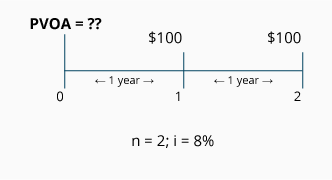Because each payment is the same amount (\$100) and because there is an equal length of time between payments (one year in this example), we know this arrangement meets the definition of an annuity. Because the equal payments occur at the end of each year, we know we have an ordinary annuity.

Calculation of Exercise #1 using the PVOA Table

The equation for calculating the present value of an ordinary annuity is: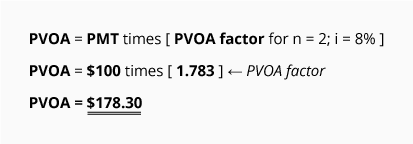This PVOA calculation tells you that receiving \$178.30 today is equivalent to receiving \$100 at the end of each of the next two years, if the time value of money is 8% per year. If the 8% rate is a company's required rate of return, this tells you that the company could pay up to \$178.30 for the two-year annuity.

[The \$178.30 could have been computed by using the PV of 1 Table for the two payments. In other words, receiving \$100 at the end of the first year has a present value of \$92.60 (\$100 times 0.926, the PV of 1 factor for n = 1; i = 8%). Receiving the second \$100 at the end of the second year has a present value of \$85.70 (\$100 times 0.857, the PV of 1 factor for n = 2; i = 8%). The total of those two present values (\$92.60 + \$85.70) equals \$178.30.]

The difference between the \$200 of total future payments and the present value of \$178.30 is the interest our money earns while we wait to receive the payments. This \$21.70 difference is referred to as interest, discount, or a company's return on its investment.

### Exercise #2

Determine the present value (the value at period 0) of receiving a series of equal payments of \$200 at the end of each year for 20 years. Assume that today is June 1, 2023 and that the first payment will occur on June 1, 2024. The appropriate rate for discounting the payments is 10% per year compounded annually.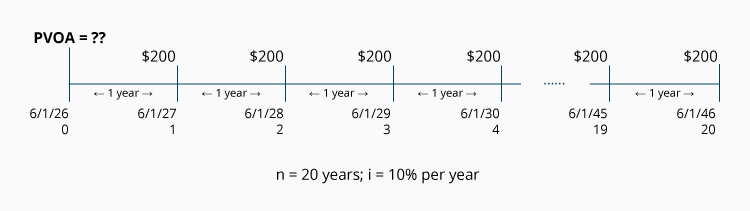Calculation of Exercise #2 using the PVOA Table

The equation for calculating the present value of an ordinary annuity is: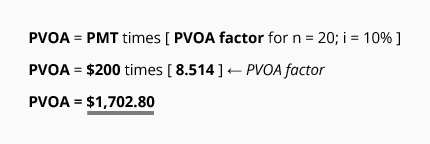This calculation tells you that receiving \$1,702.80 today is equivalent to receiving \$200 at the end of each of the next 20 years, if the time value of money is 10% per year. (Obviously, using the PVOA Table is a great time saver compared to the 20 calculations that would be required if you used the PV of 1 Table for each \$200 payment.)

Given an interest rate of 10%, the difference between the present value of \$1,702.80 and the \$4,000.00 of total payments (20 payments at \$200 each) reflects the interest earned over the years. This difference of \$2,297.20 (\$4,000 minus \$1702.80) is referred to as interest, or discount.

### Exercise #3

What is the present value of receiving a series of \$300 payments at the end of each quarter for three years, if the time value of money is 8% per year? Assume that today is June 1, 2023 and that the quarterly payments will begin on September 1, 2023.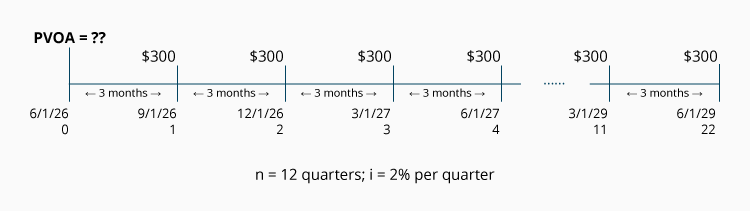As we can see from the timeline, this is an ordinary annuity; the payment amounts are identical, they occur at equal time intervals, and they occur at the end of each 3-month period.

Calculation of Exercise #3 using the PVOA Table

The equation for calculating the present value of an ordinary annuity is: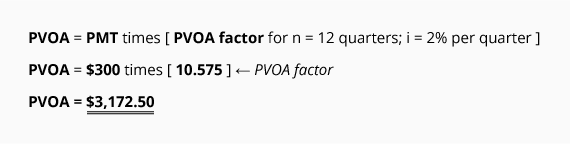This calculation tells us that receiving \$3,172.50 today is equivalent to receiving \$300 at the end of each of the next 12 quarters, if the time value of money is 2% per quarter (or 8% per year).

If 8% is a firm's targeted rate of return per year, this calculation tells us that the company can pay up to \$3,172.50 for the stream of \$300 payments. (If it pays more than \$3,172.50 it will earn less than its required rate of 8%.)

### Exercise #4

What is the maximum amount you borrow on June 1, 2023 if you are limited to a two-year loan with monthly payments of \$400? Assume the loan will have an annual interest rate of 12%. The first payment will be due on July 1, 2023.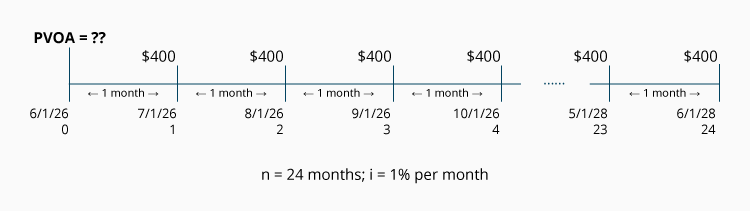Calculation of Exercise #4 using the PVOA Table

The equation for calculating the present value of an ordinary annuity is: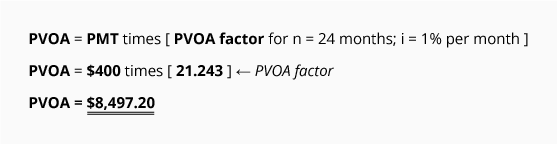Assuming the interest rate is 12% per year (or 1% per month), \$8,497.20 is the present value amount that you could borrow today if you were to make 24 monthly payments of \$400 each starting at the end of the first month. You can see the proof of this amount by reviewing the loan amortization schedule for this scenario:

Loan Amortization Schedule
(For \$8,497.20 at 12% per year with 24 monthly payments.)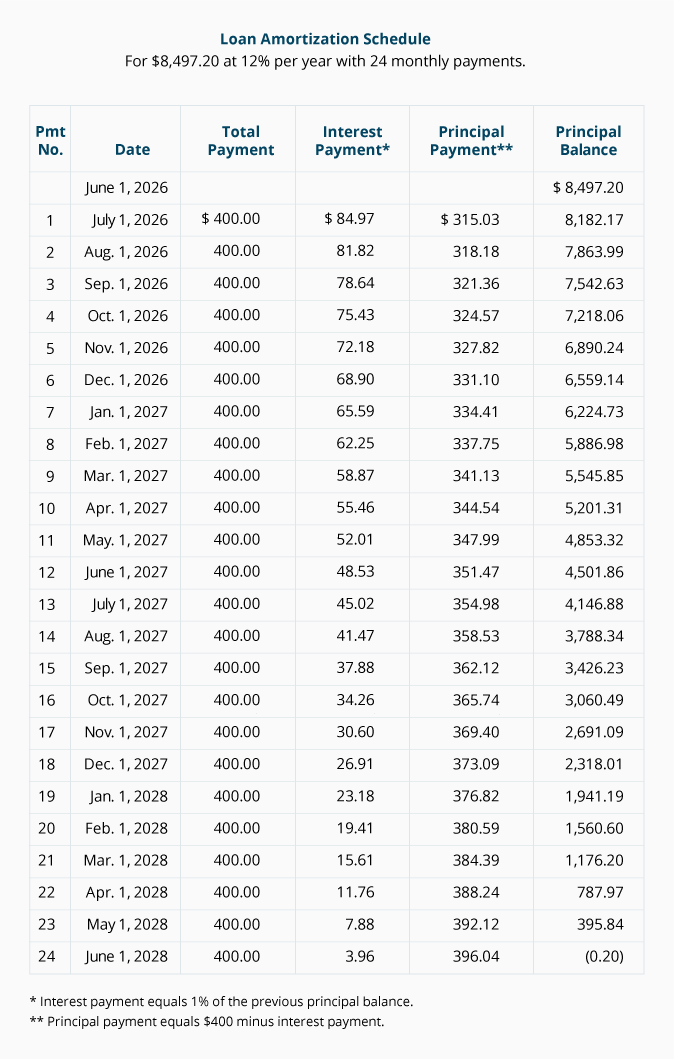The above calculation tells us that receiving \$8,497.20 today is equivalent to receiving \$400 at the end of each of the next 24 months, if the time value of money is 1% per month (or 12% per year). It also means that a company requiring a 12% annual return compounded monthly can invest up to \$8,497.20 for this annuity of \$400 payments.